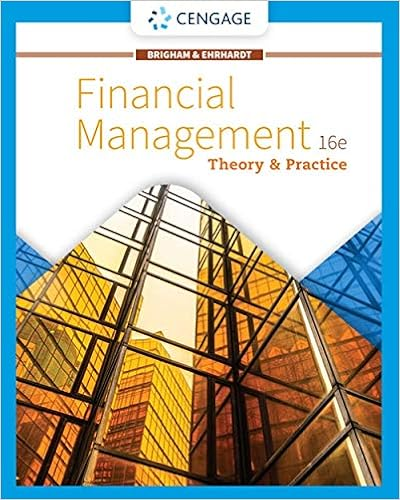# The free cash flows in millions shown below are

• 11
• 59% (17) 10 out of 17 people found this document helpful

This preview shows page 3 - 5 out of 11 pages.

##### We have textbook solutions for you!
The document you are viewing contains questions related to this textbook.The document you are viewing contains questions related to this textbook.
Chapter 21 / Exercise e
Financial Management: Theory & Practice
Brigham/EhrhardtExpert Verified
29. The free cash flows (in millions) shown below are forecast by Parker & Sons. If the weighted average cost of capital is 11% and FCF is expected to grow at a rate of 5% after Year 2, what is the Year 0 value of operations, in millions? Assume that the ROIC is expected to remain constant in Year 2 and beyond (and do not make any half-year adjustments). Year: 1 2 3
##### We have textbook solutions for you!
The document you are viewing contains questions related to this textbook.The document you are viewing contains questions related to this textbook.
Chapter 21 / Exercise e
Financial Management: Theory & Practice
Brigham/EhrhardtExpert Verified
Free cash flow: −\$50 \$100 \$1,770
30. Heath and Logan Inc. forecasts the free cash flows (in millions) shown below. The weighted average cost of capital is 13%, and the FCFs are expected to continue growing at a 5% rate after Year 3. Assuming that the ROIC is expected to remain constant in Year 3 and beyond, what is the Year 0 value of operations, in millions? Year: 1 2 3 Free cash flow: −\$15 \$10 \$40 e. \$386
31. Reynolds Construction's value of operations is \$750 million based on the free cash flow valuation model. Its balance sheet shows \$50 million of short-term investments that are unrelated to operations, \$100 million of accounts payable, \$100 million of notes payable, \$200 million of long-term debt, \$40 million of common stock (par plus paid-in-capital), and \$160 million of retained earnings. What is the best estimate for the firm's value of equity, in millions? e. \$525
32. Based on the free cash flow valuation model, the value of Weidner Co.'s operations is \$1,200 million. The company's balance sheet shows \$80 million in accounts receivable, \$60 million in inventory, and \$100 million in short-term investments that are unrelated to operations. The balance sheet also shows \$90 million in accounts payable, \$120 million in notes payable, \$300 million in long-term debt, \$50 million in preferred stock, \$180 million in retained earnings, and \$800 million in total common equity. If Weidner has 30 million shares of stock outstanding, what is the best estimate of the stock's price per share? a. \$24.90 b. \$27.67 c. \$30.43 d. \$33.48 e. \$36.82
33. The value of Broadway-Brooks Inc.'s operations is \$900 million, based on the free cash flow valuation model. Its balance sheet shows \$70 million in accounts receivable, \$50 million in inventory, \$30 million in short-term investments that are unrelated to operations, \$20 million in accounts payable, \$110 million in notes payable, \$90 million in long-term debt, \$20 million in preferred stock, \$140 million in retained earnings, and \$280 million in total common equity. If the company has 25 million shares of stock outstanding, what is the best estimate of the stock's price per share? \$34.36
•••